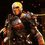# In search of the elusive answer.

Recently I came across a question which I am unable to solve. Can you guys help me?

Two tourists who are at a distance of 40 km from their camp must reach the camp together in the shortest possible time. They have one bicycle which they decide to ride in turn. One of them started walking at a speed ${ v }_{ 1 }=\quad5\quad km{ h }^{ -1 }$ and the other rode off on the bicycle at a a speed ${ v }_{ 2 }=\quad15\quad km{ h }^{ -1 }$. The tourists agreed to leave the bicycle at intermediate points between which one walks and the other rides. What is the mean speed of the tourists? How long will the bicycle remain unused?

I figured that if one tourist rides for half the distance and then walks the remaining half then both will reach the camp together. But I believe it can't be that simple. However if it is then do tell me! All solutions are welcome.Note by Siddharth Singh
7 years, 1 month ago

This discussion board is a place to discuss our Daily Challenges and the math and science related to those challenges. Explanations are more than just a solution — they should explain the steps and thinking strategies that you used to obtain the solution. Comments should further the discussion of math and science.

When posting on Brilliant:

• Use the emojis to react to an explanation, whether you're congratulating a job well done , or just really confused .
• Ask specific questions about the challenge or the steps in somebody's explanation. Well-posed questions can add a lot to the discussion, but posting "I don't understand!" doesn't help anyone.
• Try to contribute something new to the discussion, whether it is an extension, generalization or other idea related to the challenge.

MarkdownAppears as
*italics* or _italics_ italics
**bold** or __bold__ bold
- bulleted- list
• bulleted
• list
1. numbered2. list
1. numbered
2. list
Note: you must add a full line of space before and after lists for them to show up correctly
paragraph 1paragraph 2

paragraph 1

paragraph 2

[example link](https://brilliant.org)example link
> This is a quote
This is a quote
    # I indented these lines
# 4 spaces, and now they show
# up as a code block.

print "hello world"
# I indented these lines
# 4 spaces, and now they show
# up as a code block.

print "hello world"
MathAppears as
Remember to wrap math in $$ ... $$ or $ ... $ to ensure proper formatting.
2 \times 3 $2 \times 3$
2^{34} $2^{34}$
a_{i-1} $a_{i-1}$
\frac{2}{3} $\frac{2}{3}$
\sqrt{2} $\sqrt{2}$
\sum_{i=1}^3 $\sum_{i=1}^3$
\sin \theta $\sin \theta$
\boxed{123} $\boxed{123}$

Sort by:

Considering they ride and walk for equal distances (ie 20kms) they both reach the camp at the same time. Time taken by them to reach the camp would be the total time or riding the bike for 20kms at 15kmph and walking for 20kms at 5kmph. Time = distance/speed. Hence the time taken would be 4/3 hrs and 4hrs respectively. Hence the time the bicycle remains unused = 4 - 4/3 = 8/3 hrs Total time taken to reach the camp would be 4 + 4/3= 16/3 hrs Hence mean speed = distance/time =40 ÷ 16/3 = 15/2 = 7.5kmph

- 7 years, 1 month ago

So basically my reasoning was correct?

- 7 years, 1 month ago